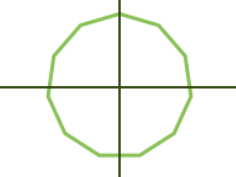Algebra

# Roots of Unity: Level 4 Challenges$\cos\left( \frac{\pi}{11}\right) - \cos\left( \frac{2\pi}{11} \right)+ \cos\left( \frac{3\pi}{11}\right) \\ - \cos\left( \frac{4\pi}{11} \right)+ \cos\left( \frac{5\pi}{11} \right)=\ ?$

Given $z^2+z+1=0,$ find the value of

$\left(z+\dfrac{1}{z} \right)^2+\left(z^2+\dfrac{1}{z^2}\right)^2+\left(z^3+\dfrac{1}{z^3} \right)^2+\cdots+\left(z^{21}+\dfrac{1}{z^{21}}\right)^2.$

$\dfrac{31-2\delta_{1}}{1-2\delta_{1}} +\dfrac{31-2\delta_{2}}{1-2\delta_{2}}+\dfrac{31-2\delta_{3}}{1-2\delta_{3}}$

If $1,\delta_{1},\delta_{2},\delta_{3}$ are distinct fourth roots of unity, then evaluate the expression above.

If $w=e^{2\pi{i}/5}$, find $\sum_{k=1}^{4}\frac{1}{1+w^k+w^{2k}}$

If for $z \in \mathbb{C} \space ,$ $z+\dfrac{1}{z}=2 \cos 6°.$ Then find the value of $\left( z^{1000}+\frac{1}{z^{1000}} \right).$

×